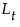smoothed level that estimatesα, the level smoothing weight
γ, the trend smoothing weight
ϕ, the trend damping weight
δ, the seasonal smoothing weight.
Each smoothing model has an ARIMA model equivalent. You may not be able to specify the equivalent ARIMA model using the ARIMA command because some smoothing models intrinsically constrain the ARIMA model parameters in ways the ARIMA command will not allow.
If we let ft=(yt+yt-1+yt-2+...yt-(k-2)+yt-(k-1))/k be the average of k consecutive observations, then:
 • for an uncentered SMA(k), ft is the plotted value for time t.
 • for a centered SMA(k) with odd k, ft is the plotted value for time t-(k-1)/2.
 • for a centered SMA(k) with even k, ft is the plotted value for time t-(k-1)/2. When saved to a data table, ft is at t-(k-2)/2.
 • for a centered SMA(k) with double smoothing for even k, the smoothing estimates are given by the following:
To fit a simple moving average model, select Smoothing > Simple Moving Average. A window appears with the following options:
Choose between No Centering, Centered, and Centered and Double Smoothed for Even Number of Terms.
The Confidence Intervals popup list allows you to set the confidence level for the forecast confidence bands. The dialogs for seasonal smoothing models include a Periods Per Season box for setting the number of periods in a season. The Constraints popup list lets you to specify what type of constraint you want to enforce on the smoothing weights during the fit. The constraints are:
expands the dialog to allow you to set constraints on individual smoothing weights. Each smoothing weight can be Bounded, Fixed, or Unconstrained as determined by the setting of the popup menu next to the weight’s name. When entering values for fixed or bounded weights, the values can be positive or negative real numbers.
The example shown here has the Level weight (α) fixed at a value of 0.3 and the Trend weight (γ) bounded by 0.1 and 0.8. In this case, the value of the Trend weight is allowed to move within the range 0.1 to 0.8 while the Level weight is held at 0.3. Note that you can specify all the smoothing weights in advance by using these custom constraints. In that case, none of the weights would be estimated from the data although forecasts and residuals would still be computed. When you click Estimate, the results of the fit appear in place of the dialog.
The smoothing equation, Lt = αyt + (1 – α)Lt-1, is defined in terms of a single smoothing weight α. This model is equivalent to an ARIMA(0, 1, 1) model where